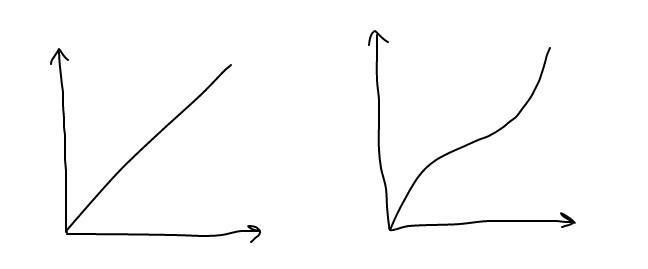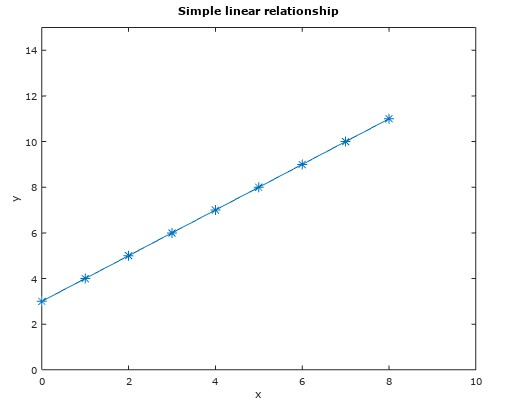Last week, we looked at TensorFlow and the work of Stanford University researchers detecting cancerous skin lesions. This week we’re going to start looking at machine learning by getting started with TensorFlow.

Imagine we have some data, and we expect it to have a linear relationship.Linear Regression is a machine learning technique that tries to find a relationship between input features, or variables, and an output. It applies when the relationship can be described as linear, and has a continuous rather than a discrete (fixed) output. For example, the relationship between the value of a car and it’s mileage might be linear and continuous: the more the mileage, the less the value.

On the other hand, classification is when the result belongs to one fixed set. For example whether or not you possess a genetic abnormality might be either “positive” or “negative”. Another classification example is whether or not a hand-written digit is a 0,1,2,3… up to 9.

## Get coding

In this example, we’re going to get stuck in straight away. We’re going to use TensorFlow to look at how to find a linear relationship between an input, a “feature”, and an output, the result. A simple example is this: we’re going to create a data set that has very basic linear mapping.

Here, we only have one input ‘`x`‘. This is transformed to get the output, ‘`y`‘:

x y
1 4
2 5
3 6
4 7
5 8
6 9
7 10
8 11Linear regression will try to work out the mapping from the input `x` to the output `y`:

`output y = weight w * input x + bias b`
`y = w*x + b`

In this example, we already know that there is a linear relationship and in this case, `y` is just `x+3`, so `w` and `b` should be:

`w = 1`
`b = 3`

## TensorFlow

TensorFlow is “an interface for expressing machine learning algorithms”. It is an open source software library, that can be used for machine learning. It was created by the Google Brain Team. A tensor refers to a typed multi-dimensional array. It is the central unit of data in TensorFlow, and is passed in between nodes. You can think of nodes as making calculations on the tensor data coming in. See Get Started for more.

### Install TensorFlow

I found the best option to be using virtualenv, as it doesn’t interfere with your existing Python environment.

Once you have activated your environment, your prompt should look like this:

`(tensorflow)\$ `

### Step-by-step

Start writing code by typing:

`python`

First we add the input data and output data, `x` and `y`.

`>>> x_data = [0,1,2,3,4,5,6,7,8]`
`>>> y_data = [3,4,5,6,7,8,9,10,11]`

Bring in the TensorFlow library:

`>>> import tensorflow as tf`

A variable is a trainable parameter in TensorFlow. They have a type and an initial value. Here, we want to randomly initialise the weight to some value between -1 and 1, and set the bias to be an array with a single element: 0. Over time, the algorithm will experiment with adjusting these values until it gets the optimum ones.

`>>> W = tf.Variable(tf.random_uniform(, -1.0, 1.0))`
`>>> b = tf.Variable(tf.zeros())`

These `tf.Variable`s are not variables in the traditional sense: they are not initialised when you call `tf.Variable`, but later when we call `sess.run(init)`.

Next, type:

`>>> y = W * x_data + b`

This is the `y = w*x + b` mapping that we are expecting. The ‘`y`‘ here corresponds to the predicted output, using the weight and bias. Remember the ‘`y_data`‘ is the “real” answer.

Below, `y - y_data` works out the difference between what the current weight and bias predict y to be, and what we know it is from the `y_data`. We then square the difference, and `reduce_mean` works out the average of all elements in the tensor. We are finding the average squared difference between the current prediction, and the actual data. This way, the algorithm can see how well it is doing, by looking at how different it’s predicted answer is to the answer it should be (the ‘cost’) across all of the example data.

`GradientDescentOptimizer` implements the gradient descent algorithm. The idea is to change the weight and bias a little bit each time. The gradient descent algorithm is a way of checking whether or not the change is reducing the cost, or increasing it. We want to reduce it, so we get closer and closer to the right answer. The value passed in is the learning rate, which impacts how big a change to make each time.

`>>> loss = tf.reduce_mean(tf.square(y - y_data))`
`>>> optimizer = tf.train.GradientDescentOptimizer(0.01)`
`>>> train = optimizer.minimize(loss)`

This (below) initialises the `tf.Variables` we created above. A Session is a class that runs the TensorFlow operations.

`>>> init = tf.global_variables_initializer()`

`>>> sess = tf.Session()`
`>>> sess.run(init)`

Below, we run the algorithm 1000 times, adjusting the weight and bias each time, and evaluating with the gradient descent algorithm. Every 10 iterations we print the values of the weight and bias: you should see them change over time to be closer to weight = 1 and bias = 3 over time.

```>>> for step in range(1000): >>>  sess.run(train) >>>  if step % 5 == 0: >>>  print(step, sess.run(W), sess.run(b))```

Next week we’ll go into more detail on linear regression. In the meantime, see the excellent resources at TensorFlow.org.

# Just Enough Code: TensorFlow and Linear Regression

| Mind the Geek| 2,137 views | 1 Comment•